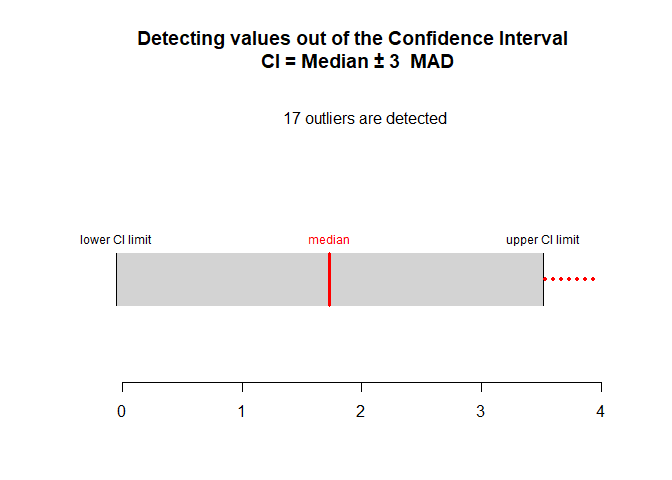# Robust Outliers Detection

Detecting outliers using robust methods, i.e. the Median Absolute Deviation (MAD) for univariate outliers; Leys, Ley, Klein, Bernard, & Licata (2013) and the Mahalanobis-Minimum Covariance Determinant (MMCD) for multivariate outliers; Leys, C., Klein, O., Dominicy, Y. & Ley, C. (2018) . There is also the more known but less robust Mahalanobis distance method, only for comparison purposes.

Routliers is designed to help researchers to detect univariate and multivariate outliers, using robust methods: - The MAD method for detecting univariate outliers (see Leys et al. 2013) - The MMCD method for detecting multivariate outliers (see Leys et al. 2018) - The Mahalanobis distance method for detecting multivariate outliers is also available in order to facilite comparisons with the MMCD method. However, this method is less robust than the MMCD method and should be avoided.

Different functions are available in order to (a) detect univariate and multivariate outliers (b) plot univariate and multivariate outliers.

## Installation

Currently, this package exists in a development version on GitHub. To use the package, you need to install it directly from GitHub using the `install_github` function from `devtools`.

You can use the following code to install the development version of `Routliers`:

As a note, one of the dependencies in `Routliers` (i.e. MASS) have its own dependencies. You may be prompted to install additional packages to be able to install `Routliers`.

## Examples of using the package

The `Routliers` package allows you to easily count, detect and plot outliers using robust methods. The most important functions in the package are `outliers_mad` and `outliers_mcd`, which allow to detect univariate and multivariate outliers, respectively. By default, the MAD function assume a normal distribution underlying the data and treat all value more than 3 MAD around the median as outliers. Moreover, the MCD function select by default h = n/2 observations on which the empirical mean and empirical covariance matrix are calculated (see Leys et al., 2018).

Here is an example of detecting extreme level of anxiety and depression (based on the HSCL-25; Derogatis, Lipman, Rickels, Uhlenhuth & Covi, 1974) in a sample of 2077 participants after the Brussels attacks, using the outliers_mad function. The 10 first items of the HSCL-25 are averaged in order to compute the level of anxiety and the 15 last items of the HSCL-25 are averaged in order to compute the level of depression. The MAD function is applied on the averaged scores.

Here is an example of detecting extremely surprising combination of scores of anxiety and depression, using the outliers_mcd function.

It is also possible to plot the outliers, using plot_outliers_mad and plot_outliers_mcd functions:the plot function for the mcd method is very useful as it allows to compare the regression line with and without outliers (showing if there is a strong impact of outliers on the regression line):# Routliers 0.0.0.9000

• Added a `NEWS.md` file to track changes to the package.

# Reference manual

install.packages("Routliers")

0.0.0.3 by Marie Delacre, a year ago

Report a bug at https://github.com/mdelacre/Routliers/issues

Browse source code at https://github.com/cran/Routliers

Authors: Marie Delacre [aut, cre] , Olivier Klein [aut]

Documentation:   PDF Manual